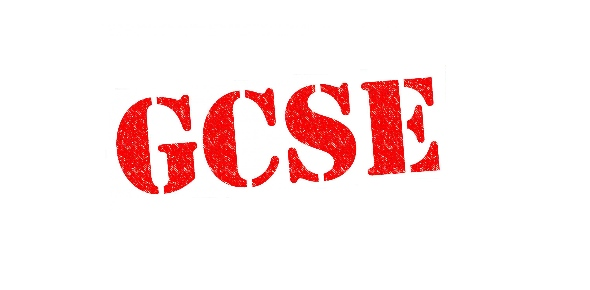# Year 10 GCSE - Spreadsheet Modelling

30 Questions | Attempts: 428
ShareSettingsAnswer all 30 questions - You cannot submit your test until you have answered all questions. All questions relate to what we covered on Wednesday and today. Best of luck! :)

• 1.
Please explain in 10 words why you should receive an A in ICT?
• 2.
A tool used to organize and analyze information
• A.

Row

• B.

• C.

Alignment

• D.

Value

• 3.
An instruction to carry out operations on the numerical data
• A.

• B.

Row

• C.

Column

• D.

Formula

• E.

Cell

• 4.
The intersection (meeting point) of a row and a column
• A.

Row

• B.

Column

• C.

Label

• D.

Cell

• 5.
What is the correct way to start a formula?
• A.

+

• B.

-

• C.

=

• D.

/

• E.

(

• 6.
What is the correct formulae for adding 2 cells together?
• A.

A1+A2

• B.

+A1/A2

• C.

=A1+A2

• D.

(A1+A2)

• E.

A1-A2

• 7.
Put this formula in the correct order (sumA1:A5=)
• A.

(=sum)A1:A5

• B.

Sum=(A1:A5)

• C.

(A1:A5)sum=

• D.

=sum(A1:A5)

• E.

=(A1:A5)sum

• 8.
What is the name of the cell in column G row 20?
• A.

02G0

• B.

20G

• C.

G20

• D.

0G2

• E.

CG20

• 9.
Spreadsheets can be used to see different scenarios to see what might happen.  What is this called?
• A.

Testing

• B.

Working Out

• C.

Estimating

• D.

Planning

• E.

Modelling

• 10.
Which symbol is used to divide to values?
• A.

-

• B.

/

• C.

=

• 11.
Which symbol is used to multiply two values?
• A.

=

• B.

*

• C.

+

• 12.
By using the cell name, such as C4, you do not have to go back into a formula and make changes when your information is changed or updated.
• A.

True

• B.

False

• 13.
What is added to the formula to create an absolute reference?
• A.

?

• B.

&

• C.

\$

• D.

#

• 14.
An example of a formula that includes and absolute reference look like?
• A.

=C12+D12+E12

• B.

=C12*D12*E12

• C.

=C12+\$B12\$

• D.

=C12= B12=D12

• 15.
If you select a cell with a date in it and drag the fill handle down several cells, what happens is _____.
• A.

The date is copied to each cell

• B.

The date is increased by one day into each cell

• C.

The date is increased by one week into each cell

• D.

Nothing happens because the fill applies only to numbers

• 16.
The formula that will add the value of D4 to the value of C2 and then multiply by the value in B2 is _____.
• A.

(D4+C2)*B2

• B.

D4+C2*B2

• C.

=D4+C2*B2

• D.

=(D4+C2)*B2

• 17.
The feature that allows you to arrange rows in alphabetical order is _____.
• A.

Sorting

• B.

Conditional formatting

• C.

Filtering

• D.

Autofill

• 18.
If a cell displays #######, it means that _____.
• A.

There is a formatting error

• B.

The column is too narrow to display all the digits of the number

• C.

There is an error in your formula

• D.

You need to center the text within the cell

• 19.
All charts use an X-axis and a Y-axis EXCEPT a _____ chart.
• A.

Bar

• B.

Line

• C.

Column

• D.

Pie

• E.

XY scatter plot

• 20.
A block of cells in an Excel worksheet is called the _____.
• A.

Area

• B.

Range

• C.

Cell reference

• D.

Name box

• E.

All of the above

• 21.
To select Cells A3-A9 and Cells D3-D9 simultaneously, you would _____.
• A.

Hold down the CTRL key, select Cells A3-A9, select Cells D3-D9

• B.

Hold down the Shift key, select Cells A3-A9, select Cells D3-D9

• C.

Select Cells A3-A9, hold down the CTRL key, select Cells D3-D9

• D.

Select Cells A3-A9, hold down the Shift key, select Cells D3-D9

• E.

Both A and B will make the selection simultaneously

• 22.
In a large spreadsheet, while scrolling down, it is helpful to make column headings visible by applying the _____ feature to the spreadsheet.
• A.

Arrange Panes

• B.

Split Panes

• C.

Freeze Panes

• D.

Conditional Formatting

• 23.
A format such as cell shading or font color that Excel can apply automatically if certain things are true is called _____.
• A.

Autoformatting

• B.

Conditional formatting

• C.

Patterns

• D.

Both a and b are correct

• 24.
A Sony digital camera price is listed as \$300.00 in Cell D5.  The digital camera discount price is listed in Cell E5 as \$20.00.  You are going to insert a formula in Cell F5 to list the final price.  Which formula is correct?
• A.

=D5+E5

• B.

=D5-E5

• C.

=E5-D5

• D.

=PRICE(D5-E5)

• 25.
To save an existing file using a different name, you would choose the _____ command.
• A.

Save

• B.

Retrieve

• C.

Save as

• D.

Store file

## Related TopicsBack to top
×

Wait!
Here's an interesting quiz for you.